# Combine Like Terms Worksheets

#### What is Combining Like Terms?

Equations and expressions, a major part of algebra, are a combination of constants, variables, arithmetic operations, and equals-to sign (only in equations). When solving equations, students very frequently come across the phrase, 'combine the like terms.' But what does it actually mean to combine the like terms? Mathematical terms that contain the same variables raised to the same power are called like terms. Combining like terms means to shorten and simplify algebraic expressions or equations so that working with them is not a hassle. To combine like terms, the coefficients Are added, and variables are kept the same.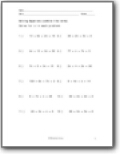###### Straight Linear Equations

Solve for x in each problem.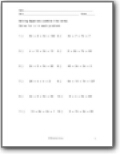###### Straight Linear Equations

Solve for x in each problem by combing like terms.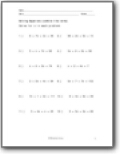###### Double Variables and Constants

Collapse all these linear equations into one.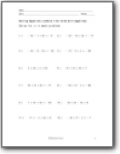###### Combine Like Terms with Negatives

Solve for x in each problem by combing like terms. Uses negative numbers.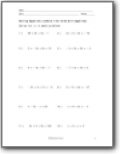###### Combine Like Terms with Negatives (Larger)

Solve for x in each problem by combing like terms. Uses negative numbers and the values are larger.